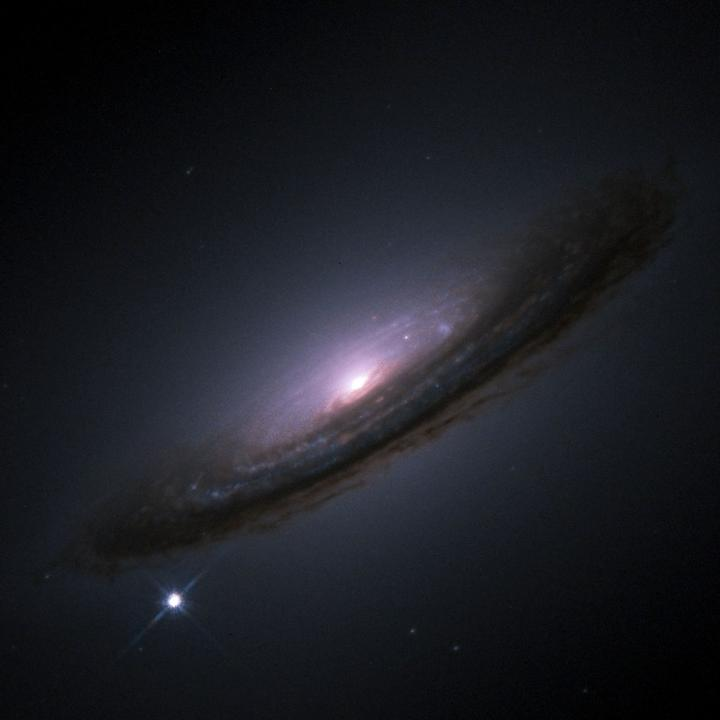# On the Hubble Constant Tension in the SNe Ia Pantheon SamplePictured is the supernova of the type Ia star 1994D, in galaxy NGC 4526. The supernova is the bright spot in the lower left corner of the image. Image credit: ESA/Hubble

### Abstract

The Hubble constant ($H_0$) tension between Type Ia Supernovae (SNe Ia) and Planck measurements ranges from 4 to 6 $\sigma$. To investigate this tension, we estimate $H_0$ in the $\Lambda$CDM and $w_0w_a$CDM models by dividing the Pantheon sample, the largest compilation of SNe Ia, into 3, 4, 20 and 40 bins. We fit the extracted $H_0$ values with a function mimicking the redshift evolution: $g(z)=H_0(z)=\tilde{H_0}/(1+z)^\alpha$, where $\alpha$ indicates an evolutionary parameter and $\tilde{H_0}=H_0$ at $z=0$. We set the absolute magnitude of SNe Ia so that $H_0=73.5\, \textrm{km}\, s^{-1} \, \textrm{Mpc}^{-1}$, and we fix fiducial values for $\Omega_{0m}^{\Lambda CDM}=0.298$ and $\Omega_{0m}^{w_0w_aCDM}=0.308$. We find that $H_0$ evolves with redshift, showing a slowly decreasing trend, with $\alpha$ coefficients consistent with zero only from 1.2 to 2.0 $\sigma$. Although the $\alpha$ coefficients are compatible with 0 in 3 $\sigma$, this however may affect cosmological results. We measure locally a variation of $H_0(z=0)-H_0(z=1)=0.4, \textrm{km}\, s^{-1} \, \textrm{Mpc}^{-1}$ in 3 and 4 bins. Extrapolating $H_0(z)$ to $z=1100$, the redshift of the last scattering surface, we obtain values of $H_0$ compatible in 1 $\sigma$ with Planck measurements independently of cosmological models and number of bins we investigated. Thus, we have reduced the $H_0$ tension from $54%$ to $72%$ for the $\Lambda$CDM and $w_0w_a$CDM models, respectively. If the decreasing trend of $H_0(z)$ is real, it could be due to astrophysical selection effects or to modified gravity.

Type
Publication
The Astrophysical Journal

Institutional press releases related to this publication:##### Enrico Rinaldi
###### Research Scientist

My research interests include artificial intelligence and quantum computing applied to particle physics and quantum many-body systems.